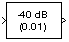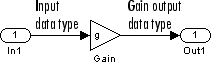# dB Gain

Apply decibel gain

## Library

Math Functions / Math Operations

`dspmathops`

•## Description

The dB Gain block multiplies the input by the decibel values specified in the Gain parameter. For an M-by-N input matrix u with elements uij, the Gain parameter can be a real M-by-N matrix with elements gij to be multiplied element-wise with the input, or a real scalar.

`${y}_{ij}={u}_{ij}{10}^{\left({g}_{ij}/k\right)}$`

The value of k is 10 for power signals (select `Power` as the Input signal parameter) and 20 for voltage signals (select `Amplitude` as the Input signal parameter).

The value of the equivalent linear gain

`${g}_{ij}^{lin}={10}^{\left({g}_{ij}/k\right)}$`

is displayed in the block icon below the dB gain value. The output is the same size as the input.

The dB Gain block supports real and complex floating-point and fixed-point data types.

### Fixed-Point Data Types

The following diagram shows the data types used within the dB Gain subsystem block for fixed-point signals.The settings for the fixed-point parameters of the Gain block in the diagram above are as follows:

• Integer rounding mode: `Floor`

• Saturate on integer overflow — unselected

• Parameter data type mode — ```Inherit via internal rule```

• Output data type mode — ```Inherit via internal rule```

## Parameters

Gain

The dB gain to apply to the input, a scalar or a real M-by-N matrix. Tunable (Simulink).

Input signal

The type of input signal: `Power` or `Amplitude`. Tunable (Simulink).

### Note

This block does not support tunability in generated code.

## Supported Data Types

• Double-precision floating point

• Single-precision floating point

• Fixed point (signed and unsigned)

• 8-, 16-, and 32-bit signed integers

• 8-, 16-, and 32-bit unsigned integers

 dB Conversion DSP System Toolbox Math Function Simulink `log10` MATLAB

Watch now